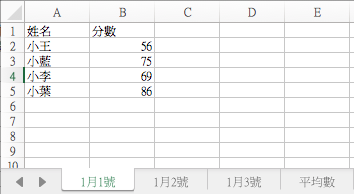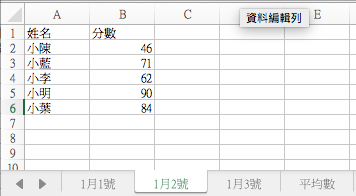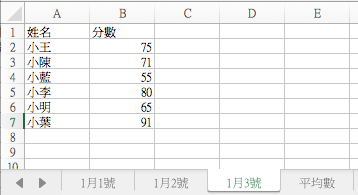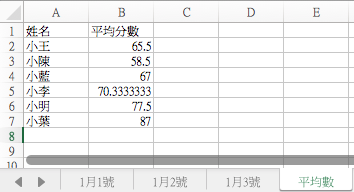0

EXCEL如何其他工作表數據並算出平均數（圖一）（圖二）（圖三）（圖四）

1 個回答

1

=COUNTIF(Sheet1!\$A\$1:\$A\$4,"="&A1)+COUNTIF(Sheet2!\$A\$1:\$A\$4,"="&A1)+COUNTIF(Sheet3!\$A\$1:\$A\$4,"="&A1)

=SUMIF(Sheet1!\$A\$1:\$A\$5,"="&A1,Sheet1!\$B\$1:\$B\$5)+SUMIF(Sheet2!\$A\$1:\$A\$5,"="&A1,Sheet2!\$B\$1:\$B\$5)+SUMIF(Sheet3!\$A\$1:\$A\$5,"="&A1,Sheet3!\$B\$1:\$B\$5)

sumif/countif= 平均值

admy6307 iT邦新手 5 級 ‧ 2017-11-08 23:28:57 檢舉